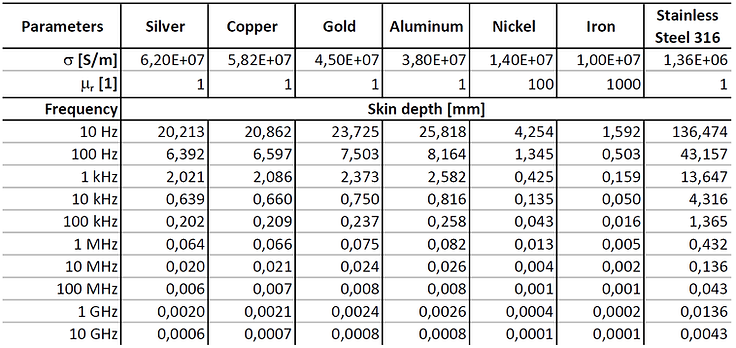top of page

## 8  Skin Effect

The resistance per unit length [Ω/m] for direct current (RDC') and for alternating current (RAC' ) of any conductor can be written as [8.1]:

ρ = specific electrical resistivity of the conductor material [Ωm]. σ = specific electrical conductivity of the conductor material [S/m]. A = cross-sectional area of the conductor [m^2], Aeff = effective cross-sectional area of the conductor through which the current effectively flows [m^2].

For direct current (DC, 0 Hz), the area A [m^2] is equal to the complete conductor diameter. However, for alternating current (AC) with frequency [Hz], the magnetic fields produced by the current in the conductor forces the current to flow towards the outer surface of the conductor. The higher the signal frequency [Hz] the smaller the cross-section Aeff [m^2] through which the current effectively flows.As a consequence of the skin effect, the resistance of a conductor increases with increasing frequency.

The diagram below shows RAC' [Ω/m] of a round copper wire with an outer diameter of D=1mm and a copper PCB trace with width w=0.25mm and height h=0.035mm (1oz). The calculations for RAC' [Ω/m] are approximations and ignore the return current path (proximity effect) and assume a single conductor surrounded only by air. However, the diagram gives an idea of how the skin effect influences the resistance at higher frequencies.Introduction and definitions of the term skin depth δ [m]:

• Conductors: The skin depth δ [m] is defined as the distance from the conductor edge where the current density has fallen to 37% (37% = 1/e = 1/2.72) of the current density at the surface of the conductor J0 [A/m^2]. The current density Jd  [A/m^2] at distance d [m] from the conductor surface is defined as [8.2]:• Shielding: Imagine an electromagnetic plane wave of field strength E0 [V/m] and H0 [A/m] entering an absorbing material (shield). The skin depth δ [m] is the distance an electromagnetic wave has to travel through that absorbing material until its field strength is reduced to 37% of E0 [V/m] or H0 [A/m] (37% = 1/e = 1/2.72). This means that the power of the plane electromagnetic wave is lowered by 20·log10(0.37) = 9dB after it traveled the distance δ [m]. The attenuation of an electromagnetic plane wave is defined like this [8.2]:Ed = remaining electric field [V/m] strength of a plane wave with field strength E0, after traveling distance d [m] through a medium with attenuation constant α [1/m]. Hd = remaining magnetic field strength [A/m] of a plane wave with field strength H0, after traveling distance d [m] through a medium with attenuation constant α [1/m]. Remember that γ = α+ is the so-called propagation constant.From above, you know that the skin depth δ [m] is defined as the inverse of the attenuation constant α [1/m] [8.3]:For good conductors (with σ>>ωε), the skin depth formula can be simplified to [8.2]:Where f [Hz] is the frequency, µr  is the relative permeability of the conductor, µ0 [H/m] is the absolute permeability and σ [S/m] is the specific electrical conductivity of the conductor material.

Below are some example values of skin depths for silver, copper, gold, aluminum, nickel, iron, and stainless steel 316. NOTE: Important to know for calculation of skin depth of nickel, iron, stainless steel, and any other ferromagnetic metal:

• Relative permeability μr  depends on the specific material and alloy (therefore, be careful when reading our table and graphic below).

• Relative permeability μr  depends on the frequency f [Hz] (neglected in the table and graphic below).bottom of page